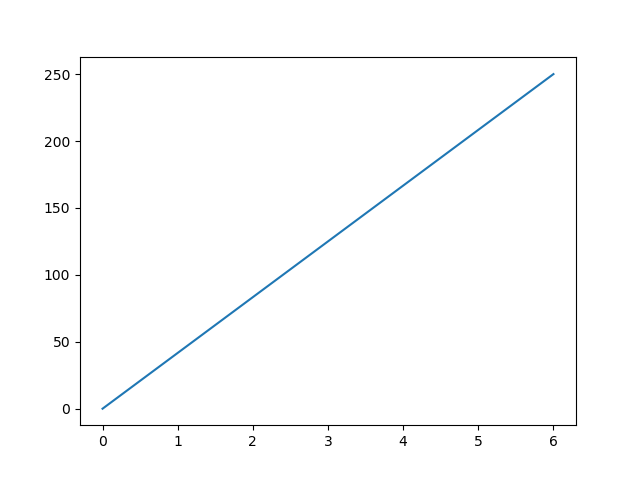Matplotlib Pyplot

Pyplot

Most of the Matplotlib utilities lies under the pyplot submodule, and are usually imported under the plt alias:

import matplotlib.pyplot as plt

Now the Pyplot package can be referred to as plt.

Example

Draw a line in a diagram from position (0,0) to position (6,250):

import matplotlib.pyplot as plt
import numpy as np

xpoints = np.array([0, 6])
ypoints = np.array([0, 250])

plt.plot(xpoints, ypoints)
plt.show()

Result:Try it Yourself »